Download PDF文章快速检索 高级检索

Application of the set-pair analysis connection number in decision-making of black-start vague set
ZHAO Yuling, ZHANG Lian
Zhejiang University of Water Resources and Electric Power, Hangzhou 310018,China
Abstract: A vague set is a kind of fuzzy set that contains uncertainty. So uncertainty analysis is required in the application of it. To solve this problem,the proposed decision-making method of a black-start vague set is based on connection number analysis of set-pair. First,rewrite the expert weights,index weights and targeted values represented by vague sets into the connection number form. Next,obtain the decision model of a vague set for black-start based on connection number,analyze the uncertainty of the model calculations,study the changes in the ranking of each project in conditions of uncertainty and select the optimal project. Practical application shows that the method can deal with the problem of optimizing black-start schemes of the correlation and uncertainty in the selected evaluation indexes effectively and clearly. It is simple in algorithm and convenient to make decision on spot.
Key words: black-start decision     vague set     correlation index     uncertainty     connection number     set-pair analysis

1 集对分析与联系数简介 1.1 集对分析

1.2 联系数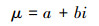(1)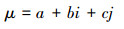2 联系数的运算

2.1 加法运算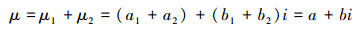2.2 乘法运算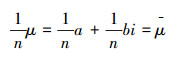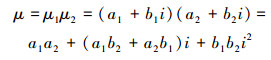(2)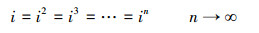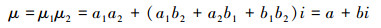3 vague集向联系数的转换 3.1 vague的概念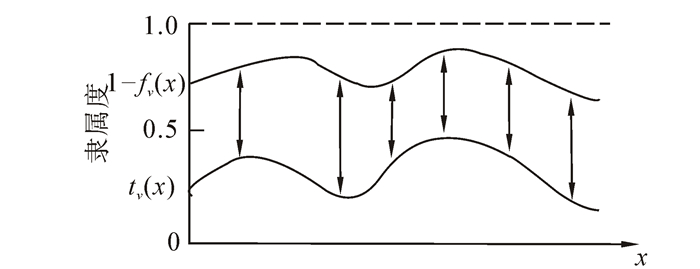图 1 Vague集 Fig. 1 Vague set

3.2 vague集的不确定度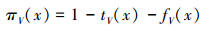(1)

πV(x)为vague集V的不确定度。

3.3 vague集的不确定性及其特征联系数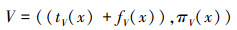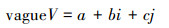(4)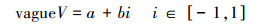(5)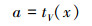(6)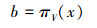(7)
4 联系数表示的黑启动决策模型 4.1 黑启动群决策的vague数据

4.1.1 黑启动方案的评价指标数据

4.1.2 指标权重W(ck)

4.1.3 专家人数与专家权重

4.2 群决策模型

4.2.1 基本模型(8)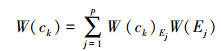(9)

Sv代表第v个方案(v=1,2,…,m)，M(Sv)表示第v个方案的综合评价值，pv(ck)表示第v个方案的指标k(k=1,2,…,n)的值，W(ck)表示指标ck的权重，W(Ej)表示专家权重。

m个方案的优劣评价准则为：M(Sv)值大的优于M(Sv)值小的。

4.2.2 关联模型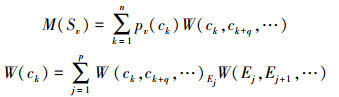4.3 专家关联权重与指标关联权重的计算

1)利用式(5)~(7)把各vague集转化为a+bi形式的联系数。

2)把Q个专家之间的关联权重W(E1,E2,…,EQ)一一折算给各个关联专家，即得平均关联权重W(E1,E2,…,EQ)的计算公式：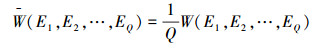3)根据上述分析得出每个专家共2p/2个平均关联权重的平均值: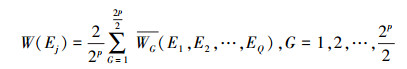4)用类似于前3步的方法计算各指标的(独立)权重。

4.4 不确定性分析

5 实例

 方案 机组状态c1 爬坡速率c2 机组容量c3 变电站个数c4 1 (0.30,0.50) (0.96,0.04) (1.00,0.00) (0.20,0.80) 2 (0.60,0.17) (0.53,0.47) (0.67,0.33) (0.25,0.75) 3 (0.45,0.33) (1.00,0.00) (0.42,0.58) (0.33,0.67) 4 (0.30,0.50) (0.50,0.50) (0.42,0.58) (0.33,0.67) 5 (0.60,0.17) (0.27,0.73) (0.42,0.58) (1.00,0.00) 6 (0.90,0.00) (0.91,0.09) (0.67,0.33) (0.25,0.75)

e3)，权重、关联权重分别为

W({e1})=(0.30,0.60)

W({e2})=(0.40,0.50)

W({e3})=(0.40,0.30)

W({e1,e2})=(0.60,0.20)

W({e1,e3})=(0.70,0.10)

W({e2,e3})=(0.70,0.20)

W({e1,e2,e3})=(1.00,0.00)

W({c1})=(0.10,0.65)

W({c2})=(0.25,0.55)

W({c3})=(0.20,0.50)

W({c4})=(0.20,0.70)

W({c1,c2})=(0.30,0.40)

W({c2,c3})=(0.50,0.25)

W({c2,c4})=(0.45,0.35)

W({c3,c4})=(0.40,0.30)

W({c1,c3})=(0.30,0.30)

W({c1,c4})=(0.30,0.40)

W({c2,c3,c4})=(0.85,0.10)

W({c1,c3,c4})=(0.70,0.20)

W({c1,c2,c4})=(0.65,0.30)

W({c1,c1,c3})=(0.75,0.20)

W({c1,c2,c3,c4})=(1.00,0.00)

1)根据式(5)～(7)计算3位专家权重如表 2

 W1) W(e2) W(e3)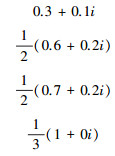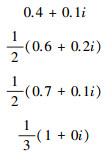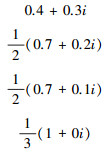平均 0.32075+0.075i 0.34575+0.0625i 0.35825+0.1125i

2)根据式(5)～(7)计算专家e1给出的黑启动指标权重及计及专家权重后的各指标权重，见表 3

 专家 指标c1 指标c2 指标c3 指标c4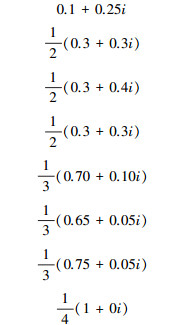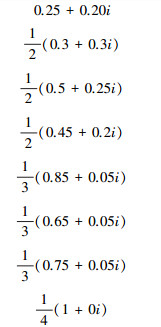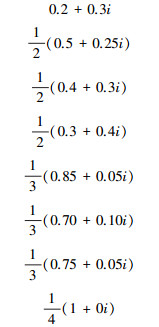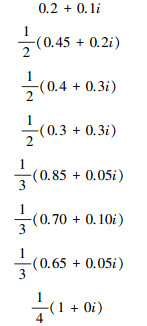平均权重联系数 (0.1875+0.1021i)× (0.32075+0.075i)= 0.0601+0.0546i (0.2344+0.0783i)× (0.32075+0.075i)= 0.0752+0.0486i (0.227+0.1053i)× (0.32075+0.075i)= 0.0728+0.0587i (0.2291+0.0709i)× (0.32075+0.075i)= 0.0735+0.0452i

W(c1)=0.2034+0.1877i

W(c2)=0.2116+0.1732i

W(c3)=0.2144+0.1797i

W(c4)=0.2255+0.1702i

3)把表 1中的各vague集数据改写成联系数，得表 4

 方案 c1 c2 c3 c4 1 0.30+0.20i 0.96+0i 1+0i 0.2+0i 2 0.60+0.23i 0.53+0i 0.67+0i 0.25+0i 3 0.45+0.22i 1+0i 0.42+0i 0.33+0i 4 0.30+0.20i 0.50+0i 0.42+0i 0.33+0i 5 0.60+0.23i 0.27+0i 0.42+0i 1+0i 6 0.90+0.10i 0.91+0i 0.67+0i 0.25+0i

4)利用表 4，并结合第2步得到的各指标权重，采用式(8)算得各方案的综合评价值联系数为

M(s1)=0.523 62+0.514 542i

M(s2)=0.434 211+0.457 318i

M(s3)=0.467 593+0.475 347i

M(s4)=0.331 283+0.352 77i

M(s5)=0.494 72+0.495 011i

M(s6)=0.575 639+0.528 601i

5)对各方案的综合评价值联系数作不确定性计算分析，得表 5

 i=－1 i=－0.5 i=0 i=0.5 i=1 M(s1) 0.0091② 0.2663② 0.5237② 0.7809② 1.0382② M(s2) -0.0231⑥ 0.2056⑤ 0.5237② 0.6629⑤ 0.8915⑤ M(s3) -0.0078④ 0.2299④ 0.4676④ 0.7053④ 0.9429④ M(s4) -0.0215⑤ 0.1549⑥ 0.3313⑥ 0.5077⑥ 0.6840⑥ M(s5) -0.0002③ 0.2472③ 0.4947③ 0.7422③ 0.9897③ M(s6) 0.0470① 0.3113① 0.5756① 0.8299① 1.1042①

 方案 第1次 第2次 第3次 第4次 第5次 第6次 总分 1 16 18 8 12 8 2 272 2 2 8 12 8 3 31 161 3 4 12 12 12 16 8 208 4 1 5 10 10 20 18 159 5 8 16 12 12 12 4 240 6 33 5 10 10 5 1 304 Σ 64 64 64 64 64 64 1344

6 结束语

W({c1})=(0.15,0.7)

W({c2})=(0.2,0.7)

W({c3})=(0.15,0.7)

W({c4})=(0.25,0.55)

W({c1,c2})=(0.3,0.5)

W({c2,c3})=(0.37,0.43)

W({c2,c4})=(0.5,0.3)

W({c3,c4})=(0.45,0.3)

W({c1,c3})=(0.35,0.45)

W({c1,c4})=(0.45,0.30)

W({c2,c3,c4})=(0.75,0.10)

W({c1,c3,c4})=(0.75,0.10)

W({c1,c2,c4})=(0.68,0.15)

W({c1,c2,c3})=(0.55,0.25)

W({c1,c2,c3,c4})=(1.00,0.00)

W({c1})=(0.2,0.5)

W({c2})=(0.1,0.65)

W({c3})=(0.15,0.7)

W({c4})=(0.15,0.7)

W({c1,c2})=(0.35,0.3)

W({c2,c3})=(0.3,0.4)

W({c2,c4})=(0.3,0.4)

W({c3,c4})=(0.35,0.4)

W({c1,c3})=(0.42,0.28)

W({c1,c4})=(0.42,0.28)

W(c2,c3,c4)=(0.6,0.05)

W(c1,c3,c4)=(0.65,0.05)

W(c1,c2,c4)=(0.55,0.15)

W(c1,c2,c3)=(0.6,0.05)

W(c1,c2,c3,c4)=(1.00,0.00)

 专家 指标c1 指标c2 指标c3 指标c4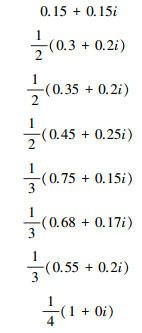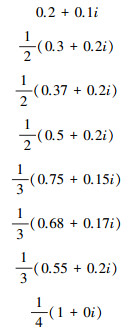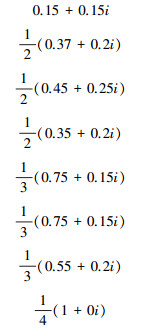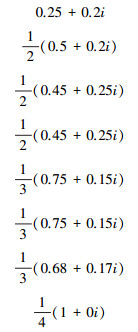平均权重联系数 (0.2013+0.0810i)×(0.34575+0.0625i)=0.0696+0.0457i (0.2119+0.0716i)×(0.34575+0.0625i)=0.0733+0.0425i (0.2085+0.0802i)×(0.34575+0.0625i)=0.0721+0.0457i (0.2409+0.0883i)×(0.34575+0.0625i)=0.0833+0.0510i

 专家 指标c1 指标c2 指标c3 指标c4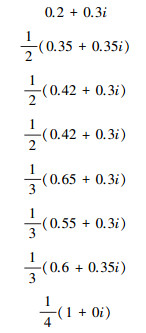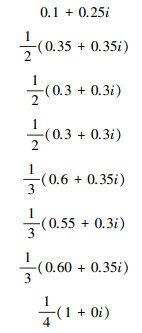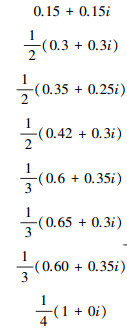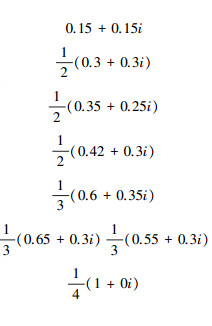平均权重联系数 (0.2056+0.1365i)×(0.35825+0.1125i)=0.0737+0.0874i (0.1764+0.1324i)×(0.35825+0.1125i)=0.0631+0.0821i (0.194+0.113i)×(0.35825+0.1125i)=0.0695+0.0753i (0.1910+0.1115i)×(0.35825+0.1125i)=0.0687+0.0740i

   THALE S,AGARWAL V. A smart control strategy for the black start of a microgrid based on PV and other auxiliary sources under islanded condition[C]//37th IEEE Photovoltaic Specialists Conference. [S.1]:IEEE,2011:2454-2459.  ADIBI M M, KAFKA L R J, MILANICZ D P. Expert system requirements for power system restoration[J]. IEEE Trans on Power Systems , 1994, 9 (3) : 1592-1598  林济铿, 蒋越梅, 岳顺民, 等. 基于DEA/AHP模型的电力系统黑启动有效方案评估[J]. 电力系统自动化 , 2007, 31 (15) : 65-69 LIN Jikeng, JIANG Yuemei, YUE Shunmin, et al. Assessment of effective schemes for power system blackstart based on EDA/AHP[J]. Automation of Electric Power Systems , 2007, 31 (15) : 65-69  ISLAM S, CHOWDHURY N. A case-base windows graphic package for the education and training of power system restoration[J]. IEEE Trans on Power Systems , 2001, 16 (2) : 181-187  刘伟佳, 林振智, 文福拴, 等. 基于直觉模糊距离的黑启动群体决策一致性分析与优化[J]. 电力系统自动化 , 2012, 36 (10) : 1-6 LIU Weijia, LIN Zhenzhi, WEN Fushuan, et al. Consistency analysis and optimization of black-start decision consistency based on intutionistic fuzzy distance[J]. Automation of Electric Power Systems , 2012, 36 (10) : 1-6  曾顺奇, 林振智, 文福拴, 等. 基于vague集理论的黑启动决策模型与方法[J]. 电力系统自动化 , 2011, 35 (2) : 18-22 ZENG Shunqi, LIN Zhenzhi, WEN Fushuan, et al. The model and method of black-start decision based on the theory of vague set[J]. Automation of Electric Power Systems , 2011, 35 (2) : 18-22  赵克勤. 集对分析及其初步应用[M]. 杭州: 浙江科学技术出版社, 2000 : 1 -18.  赵克勤. 二元联系数A+Bi的理论基础和基本算法及在人工智能中的应用[J]. 智能系统学报 , 2008, 3 (6) : 16-22  ZHAO Keqin. The two element connection number A+Bi basic theory and algorithm and its application in artificial intelligence[J]. CAAI Transactions on Intelligent Systems , 2008, 3 (6) : 16-22  金华征, 程浩忠, 杨晓梅, 等. 基于联系数模型的电网灵活规划方法[J]. 中国电机工程学报 , 2006, 43 (12) : 16-20 JIN Huazheng, CHENG Haozhong, YANG Xiaomei, et al. The method of transmission network flexible planning based on the connection number model[J]. Proceedings of the CSEE , 2006, 43 (12) : 16-20  万官泉, 张尧, 汪穗峰. 基于联系数的配电系统可靠性不确定性评估[J]. 电力系统自动化 , 2008, 32 (4) : 31-34 WAN Guanquan, ZHANG Yao, WANG Suifeng. Uncertainty evaluation for distribution system reliability based on connection number[J]. Automation of Electric Power Systems , 2008, 32 (4) : 31-34  陈卫东, 肖先勇, 陈礼频, 等. 考虑可靠性参数影响的电压暂降频次联系数评估方法[J]. 中国电机工程学报 , 2010, 47 (31) : 35-42 CHEN Weidong, XIAO Xianyong, CHEN Lipin, et al. Connection number evaluation method of the voltage sag frequency considered reliability parameters affection[J]. Proceedings of the CSEE , 2010, 47 (31) : 35-42  安磊, 王绵斌, 谭忠富. 基于集对故障树法的输变电工程风险评估模型[J]. 华东电力 , 2011, 40 (1) : 12-18 AN Lei, WANG Mianbin, TAN Zhongfu. The assessment model of power transmission project risk based on fault tree analysis method[J]. East China Electric Power , 2011, 40 (1) : 12-18  刘俊华, 罗隆福, 张志文, 等. 基于模糊集对分析法的电能质量综合评价[J]. 电网技术 , 2012, 36 (7) : 81-85 LIU Junhua, LUO Longfu, ZHANG Zhiwen, et al. Comprehensive evaluation of power quality based on fuzzy set pair analysis[J]. Power System Technology , 2012, 36 (7) : 81-85  刘俊华, 罗隆福, 张志文, 等. 一种考虑排序稳定分析的电能质量综合评估新方法[J]. 中国电机工程学报 , 2013, 33 (1) : 70-76 LIU Junhua, LUO Longfu, ZHANG Zhiwen, et al. A new method for power quality comprehensive evaluation considering the analysis of sequence stability[J]. Proceedings of the CSEE , 2013, 33 (1) : 70-76  刘秀梅, 赵克勤. 基于集对分析联系数的信息不完全直觉模糊多属性决策[J]. 数学的实践与认识 , 2010, 40 (1) : 67-77 LIU Xiumei, ZHAO Keqin. Multiple attribute decision making with the incomplete information and intuitionistic fuzzy number based on the connection number of set-pair analysis[J]. Mathematics in Practice and Theory , 2010, 40 (1) : 67-77  BUSTINCE H, BURILLO P. vague sets are intuitionistic fuzzy sets[J]. Fuzzy Sets and Systems , 1996, 79 (3) : 403-405  赵克勤. 集对分析的不确定性系统理论在人工智能中的应用[J]. 智能系统学报 , 2006, 1 (2) : 18-25 ZHAO Keqin. Application in artificial intelligence of uncertainty system theory of set-pair analysis[J]. CAAI Transactions on Intelligent Systems , 2006, 1 (2) : 18-25  赵克勤. 基于集对分析的不确定性多属性决策模型与算法[J]. 智能系统学报 , 2010, 5 (1) : 41-50 ZHAO Keqin. Application in artificial intelligence of uncertainty system theory of set-pair analysis[J]. CAAI Transactions on Intelligent Systems , 2010, 5 (1) : 41-50
DOI: 10.3969/j.issn.1673-4785.201305002

0

#### 文章信息

ZHAO Yuling, ZHANG Lian

Application of the set-pair analysis connection number in decision-making of black-start vague set

CAAI Transactions on Intelligent Systems, 2014, 9(5): 632-640
http://dx.doi.org/10.3969/j.issn.1673-4785.201305002